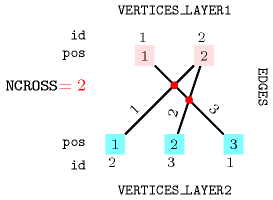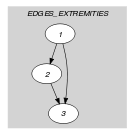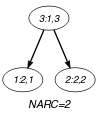## 5.407. two_layer_edge_crossing

Origin

Inspired by [HararySchwenk72].

Constraint

$\mathrm{𝚝𝚠𝚘}_\mathrm{𝚕𝚊𝚢𝚎𝚛}_\mathrm{𝚎𝚍𝚐𝚎}_\mathrm{𝚌𝚛𝚘𝚜𝚜𝚒𝚗𝚐}\left(\begin{array}{c}\mathrm{𝙽𝙲𝚁𝙾𝚂𝚂},\hfill \\ \mathrm{𝚅𝙴𝚁𝚃𝙸𝙲𝙴𝚂}_\mathrm{𝙻𝙰𝚈𝙴𝚁}\mathtt{1},\hfill \\ \mathrm{𝚅𝙴𝚁𝚃𝙸𝙲𝙴𝚂}_\mathrm{𝙻𝙰𝚈𝙴𝚁}\mathtt{2},\hfill \\ \mathrm{𝙴𝙳𝙶𝙴𝚂}\hfill \end{array}\right)$

Arguments
 $\mathrm{𝙽𝙲𝚁𝙾𝚂𝚂}$ $\mathrm{𝚍𝚟𝚊𝚛}$ $\mathrm{𝚅𝙴𝚁𝚃𝙸𝙲𝙴𝚂}_\mathrm{𝙻𝙰𝚈𝙴𝚁}\mathtt{1}$ $\mathrm{𝚌𝚘𝚕𝚕𝚎𝚌𝚝𝚒𝚘𝚗}\left(\mathrm{𝚒𝚍}-\mathrm{𝚒𝚗𝚝},\mathrm{𝚙𝚘𝚜}-\mathrm{𝚍𝚟𝚊𝚛}\right)$ $\mathrm{𝚅𝙴𝚁𝚃𝙸𝙲𝙴𝚂}_\mathrm{𝙻𝙰𝚈𝙴𝚁}\mathtt{2}$ $\mathrm{𝚌𝚘𝚕𝚕𝚎𝚌𝚝𝚒𝚘𝚗}\left(\mathrm{𝚒𝚍}-\mathrm{𝚒𝚗𝚝},\mathrm{𝚙𝚘𝚜}-\mathrm{𝚍𝚟𝚊𝚛}\right)$ $\mathrm{𝙴𝙳𝙶𝙴𝚂}$ $\mathrm{𝚌𝚘𝚕𝚕𝚎𝚌𝚝𝚒𝚘𝚗}\left(\mathrm{𝚒𝚍}-\mathrm{𝚒𝚗𝚝},\mathrm{𝚟𝚎𝚛𝚝𝚎𝚡}\mathtt{1}-\mathrm{𝚒𝚗𝚝},\mathrm{𝚟𝚎𝚛𝚝𝚎𝚡}\mathtt{2}-\mathrm{𝚒𝚗𝚝}\right)$
Restrictions
 $\mathrm{𝙽𝙲𝚁𝙾𝚂𝚂}\ge 0$ $\mathrm{𝚛𝚎𝚚𝚞𝚒𝚛𝚎𝚍}$$\left(\mathrm{𝚅𝙴𝚁𝚃𝙸𝙲𝙴𝚂}_\mathrm{𝙻𝙰𝚈𝙴𝚁}\mathtt{1},\left[\mathrm{𝚒𝚍},\mathrm{𝚙𝚘𝚜}\right]\right)$ $\mathrm{𝚅𝙴𝚁𝚃𝙸𝙲𝙴𝚂}_\mathrm{𝙻𝙰𝚈𝙴𝚁}\mathtt{1}.\mathrm{𝚒𝚍}\ge 1$ $\mathrm{𝚅𝙴𝚁𝚃𝙸𝙲𝙴𝚂}_\mathrm{𝙻𝙰𝚈𝙴𝚁}\mathtt{1}.\mathrm{𝚒𝚍}\le |\mathrm{𝚅𝙴𝚁𝚃𝙸𝙲𝙴𝚂}_\mathrm{𝙻𝙰𝚈𝙴𝚁}\mathtt{1}|$ $\mathrm{𝚍𝚒𝚜𝚝𝚒𝚗𝚌𝚝}$$\left(\mathrm{𝚅𝙴𝚁𝚃𝙸𝙲𝙴𝚂}_\mathrm{𝙻𝙰𝚈𝙴𝚁}\mathtt{1},\mathrm{𝚒𝚍}\right)$ $\mathrm{𝚍𝚒𝚜𝚝𝚒𝚗𝚌𝚝}$$\left(\mathrm{𝚅𝙴𝚁𝚃𝙸𝙲𝙴𝚂}_\mathrm{𝙻𝙰𝚈𝙴𝚁}\mathtt{1},\mathrm{𝚙𝚘𝚜}\right)$ $\mathrm{𝚛𝚎𝚚𝚞𝚒𝚛𝚎𝚍}$$\left(\mathrm{𝚅𝙴𝚁𝚃𝙸𝙲𝙴𝚂}_\mathrm{𝙻𝙰𝚈𝙴𝚁}\mathtt{2},\left[\mathrm{𝚒𝚍},\mathrm{𝚙𝚘𝚜}\right]\right)$ $\mathrm{𝚅𝙴𝚁𝚃𝙸𝙲𝙴𝚂}_\mathrm{𝙻𝙰𝚈𝙴𝚁}\mathtt{2}.\mathrm{𝚒𝚍}\ge 1$ $\mathrm{𝚅𝙴𝚁𝚃𝙸𝙲𝙴𝚂}_\mathrm{𝙻𝙰𝚈𝙴𝚁}\mathtt{2}.\mathrm{𝚒𝚍}\le |\mathrm{𝚅𝙴𝚁𝚃𝙸𝙲𝙴𝚂}_\mathrm{𝙻𝙰𝚈𝙴𝚁}\mathtt{2}|$ $\mathrm{𝚍𝚒𝚜𝚝𝚒𝚗𝚌𝚝}$$\left(\mathrm{𝚅𝙴𝚁𝚃𝙸𝙲𝙴𝚂}_\mathrm{𝙻𝙰𝚈𝙴𝚁}\mathtt{2},\mathrm{𝚒𝚍}\right)$ $\mathrm{𝚍𝚒𝚜𝚝𝚒𝚗𝚌𝚝}$$\left(\mathrm{𝚅𝙴𝚁𝚃𝙸𝙲𝙴𝚂}_\mathrm{𝙻𝙰𝚈𝙴𝚁}\mathtt{2},\mathrm{𝚙𝚘𝚜}\right)$ $\mathrm{𝚛𝚎𝚚𝚞𝚒𝚛𝚎𝚍}$$\left(\mathrm{𝙴𝙳𝙶𝙴𝚂},\left[\mathrm{𝚒𝚍},\mathrm{𝚟𝚎𝚛𝚝𝚎𝚡}\mathtt{1},\mathrm{𝚟𝚎𝚛𝚝𝚎𝚡}\mathtt{2}\right]\right)$ $\mathrm{𝙴𝙳𝙶𝙴𝚂}.\mathrm{𝚒𝚍}\ge 1$ $\mathrm{𝙴𝙳𝙶𝙴𝚂}.\mathrm{𝚒𝚍}\le |\mathrm{𝙴𝙳𝙶𝙴𝚂}|$ $\mathrm{𝚍𝚒𝚜𝚝𝚒𝚗𝚌𝚝}$$\left(\mathrm{𝙴𝙳𝙶𝙴𝚂},\mathrm{𝚒𝚍}\right)$ $\mathrm{𝙴𝙳𝙶𝙴𝚂}.\mathrm{𝚟𝚎𝚛𝚝𝚎𝚡}\mathtt{1}\ge 1$ $\mathrm{𝙴𝙳𝙶𝙴𝚂}.\mathrm{𝚟𝚎𝚛𝚝𝚎𝚡}\mathtt{1}\le |\mathrm{𝚅𝙴𝚁𝚃𝙸𝙲𝙴𝚂}_\mathrm{𝙻𝙰𝚈𝙴𝚁}\mathtt{1}|$ $\mathrm{𝙴𝙳𝙶𝙴𝚂}.\mathrm{𝚟𝚎𝚛𝚝𝚎𝚡}\mathtt{2}\ge 1$ $\mathrm{𝙴𝙳𝙶𝙴𝚂}.\mathrm{𝚟𝚎𝚛𝚝𝚎𝚡}\mathtt{2}\le |\mathrm{𝚅𝙴𝚁𝚃𝙸𝙲𝙴𝚂}_\mathrm{𝙻𝙰𝚈𝙴𝚁}\mathtt{2}|$
Purpose

$\mathrm{𝙽𝙲𝚁𝙾𝚂𝚂}$ is the number of line segments intersections.

Example
$\left(\begin{array}{c}2,〈\mathrm{𝚒𝚍}-1\mathrm{𝚙𝚘𝚜}-1,\mathrm{𝚒𝚍}-2\mathrm{𝚙𝚘𝚜}-2〉,\hfill \\ 〈\mathrm{𝚒𝚍}-1\mathrm{𝚙𝚘𝚜}-3,\mathrm{𝚒𝚍}-2\mathrm{𝚙𝚘𝚜}-1,\mathrm{𝚒𝚍}-3\mathrm{𝚙𝚘𝚜}-2〉,\hfill \\ 〈\begin{array}{ccc}\mathrm{𝚒𝚍}-1\hfill & \mathrm{𝚟𝚎𝚛𝚝𝚎𝚡}\mathtt{1}-2\hfill & \mathrm{𝚟𝚎𝚛𝚝𝚎𝚡}\mathtt{2}-2,\hfill \\ \mathrm{𝚒𝚍}-2\hfill & \mathrm{𝚟𝚎𝚛𝚝𝚎𝚡}\mathtt{1}-2\hfill & \mathrm{𝚟𝚎𝚛𝚝𝚎𝚡}\mathtt{2}-3,\hfill \\ \mathrm{𝚒𝚍}-3\hfill & \mathrm{𝚟𝚎𝚛𝚝𝚎𝚡}\mathtt{1}-1\hfill & \mathrm{𝚟𝚎𝚛𝚝𝚎𝚡}\mathtt{2}-1\hfill \end{array}〉\hfill \end{array}\right)$

Figure 5.407.1 provides a picture of the example, where one can see the two line segments intersections. Each line segment of Figure 5.407.1 is labelled with its identifier and corresponds to an item of the $\mathrm{𝙴𝙳𝙶𝙴𝚂}$ collection. The two vertices on top of Figure 5.407.1 correspond to the items of the $\mathrm{𝚅𝙴𝚁𝚃𝙸𝙲𝙴𝚂}_\mathrm{𝙻𝙰𝚈𝙴𝚁}\mathtt{1}$ collection, while the three other vertices are associated with the items of $\mathrm{𝚅𝙴𝚁𝚃𝙸𝙲𝙴𝚂}_\mathrm{𝙻𝙰𝚈𝙴𝚁}\mathtt{2}$.

##### Figure 5.407.1. Intersection between line segments joining two layers of the Example slot for the constraint $\mathrm{𝚝𝚠𝚘}_\mathrm{𝚕𝚊𝚢𝚎𝚛}_\mathrm{𝚎𝚍𝚐𝚎}_\mathrm{𝚌𝚛𝚘𝚜𝚜𝚒𝚗𝚐}$$\left(\mathrm{𝙽𝙲𝚁𝙾𝚂𝚂},\mathrm{𝚅𝙴𝚁𝚃𝙸𝙲𝙴𝚂}_\mathrm{𝙻𝙰𝚈𝙴𝚁}\mathtt{1},\mathrm{𝚅𝙴𝚁𝚃𝙸𝙲𝙴𝚂}_\mathrm{𝙻𝙰𝚈𝙴𝚁}\mathtt{2},\mathrm{𝙴𝙳𝙶𝙴𝚂}\right)$Typical
 $|\mathrm{𝚅𝙴𝚁𝚃𝙸𝙲𝙴𝚂}_\mathrm{𝙻𝙰𝚈𝙴𝚁}\mathtt{1}|>1$ $|\mathrm{𝚅𝙴𝚁𝚃𝙸𝙲𝙴𝚂}_\mathrm{𝙻𝙰𝚈𝙴𝚁}\mathtt{2}|>1$ $|\mathrm{𝙴𝙳𝙶𝙴𝚂}|\ge |\mathrm{𝚅𝙴𝚁𝚃𝙸𝙲𝙴𝚂}_\mathrm{𝙻𝙰𝚈𝙴𝚁}\mathtt{1}|$ $|\mathrm{𝙴𝙳𝙶𝙴𝚂}|\ge |\mathrm{𝚅𝙴𝚁𝚃𝙸𝙲𝙴𝚂}_\mathrm{𝙻𝙰𝚈𝙴𝚁}\mathtt{2}|$
Symmetries
• Arguments are permutable w.r.t. permutation $\left(\mathrm{𝙽𝙲𝚁𝙾𝚂𝚂}\right)$ $\left(\mathrm{𝚅𝙴𝚁𝚃𝙸𝙲𝙴𝚂}_\mathrm{𝙻𝙰𝚈𝙴𝚁}\mathtt{1},\mathrm{𝚅𝙴𝚁𝚃𝙸𝙲𝙴𝚂}_\mathrm{𝙻𝙰𝚈𝙴𝚁}\mathtt{2}\right)$ $\left(\mathrm{𝙴𝙳𝙶𝙴𝚂}\right)$.

• Items of $\mathrm{𝚅𝙴𝚁𝚃𝙸𝙲𝙴𝚂}_\mathrm{𝙻𝙰𝚈𝙴𝚁}\mathtt{1}$ are permutable.

• Items of $\mathrm{𝚅𝙴𝚁𝚃𝙸𝙲𝙴𝚂}_\mathrm{𝙻𝙰𝚈𝙴𝚁}\mathtt{2}$ are permutable.

Arg. properties

Functional dependency: $\mathrm{𝙽𝙲𝚁𝙾𝚂𝚂}$ determined by $\mathrm{𝚅𝙴𝚁𝚃𝙸𝙲𝙴𝚂}_\mathrm{𝙻𝙰𝚈𝙴𝚁}\mathtt{1}$, $\mathrm{𝚅𝙴𝚁𝚃𝙸𝙲𝙴𝚂}_\mathrm{𝙻𝙰𝚈𝙴𝚁}\mathtt{2}$ and $\mathrm{𝙴𝙳𝙶𝙴𝚂}$.

Remark

The two-layer edge crossing minimisation problem was proved to be NP-hard in [GareyJohnson83].

Keywords
Derived Collection
$\mathrm{𝚌𝚘𝚕}\left(\begin{array}{c}\mathrm{𝙴𝙳𝙶𝙴𝚂}_\mathrm{𝙴𝚇𝚃𝚁𝙴𝙼𝙸𝚃𝙸𝙴𝚂}-\mathrm{𝚌𝚘𝚕𝚕𝚎𝚌𝚝𝚒𝚘𝚗}\left(\mathrm{𝚕𝚊𝚢𝚎𝚛}\mathtt{1}-\mathrm{𝚍𝚟𝚊𝚛},\mathrm{𝚕𝚊𝚢𝚎𝚛}\mathtt{2}-\mathrm{𝚍𝚟𝚊𝚛}\right),\hfill \\ \left[\begin{array}{c}\mathrm{𝚒𝚝𝚎𝚖}\left(\begin{array}{c}\mathrm{𝚕𝚊𝚢𝚎𝚛}\mathtt{1}-\mathrm{𝙴𝙳𝙶𝙴𝚂}.\mathrm{𝚟𝚎𝚛𝚝𝚎𝚡}\mathtt{1}\left(\mathrm{𝚅𝙴𝚁𝚃𝙸𝙲𝙴𝚂}_\mathrm{𝙻𝙰𝚈𝙴𝚁}\mathtt{1},\mathrm{𝚙𝚘𝚜},\mathrm{𝚒𝚍}\right),\hfill \\ \mathrm{𝚕𝚊𝚢𝚎𝚛}\mathtt{2}-\mathrm{𝙴𝙳𝙶𝙴𝚂}.\mathrm{𝚟𝚎𝚛𝚝𝚎𝚡}\mathtt{2}\left(\mathrm{𝚅𝙴𝚁𝚃𝙸𝙲𝙴𝚂}_\mathrm{𝙻𝙰𝚈𝙴𝚁}\mathtt{2},\mathrm{𝚙𝚘𝚜},\mathrm{𝚒𝚍}\right)\hfill \end{array}\right)\hfill \end{array}\right]\hfill \end{array}\right)$
Arc input(s)

$\mathrm{𝙴𝙳𝙶𝙴𝚂}_\mathrm{𝙴𝚇𝚃𝚁𝙴𝙼𝙸𝚃𝙸𝙴𝚂}$

Arc generator
$\mathrm{𝐶𝐿𝐼𝑄𝑈𝐸}$$\left(<\right)↦\mathrm{𝚌𝚘𝚕𝚕𝚎𝚌𝚝𝚒𝚘𝚗}\left(\mathrm{𝚎𝚍𝚐𝚎𝚜}_\mathrm{𝚎𝚡𝚝𝚛𝚎𝚖𝚒𝚝𝚒𝚎𝚜}\mathtt{1},\mathrm{𝚎𝚍𝚐𝚎𝚜}_\mathrm{𝚎𝚡𝚝𝚛𝚎𝚖𝚒𝚝𝚒𝚎𝚜}\mathtt{2}\right)$

Arc arity
Arc constraint(s)
$\bigvee \left(\begin{array}{c}\bigwedge \left(\begin{array}{c}\mathrm{𝚎𝚍𝚐𝚎𝚜}_\mathrm{𝚎𝚡𝚝𝚛𝚎𝚖𝚒𝚝𝚒𝚎𝚜}\mathtt{1}.\mathrm{𝚕𝚊𝚢𝚎𝚛}\mathtt{1}<\mathrm{𝚎𝚍𝚐𝚎𝚜}_\mathrm{𝚎𝚡𝚝𝚛𝚎𝚖𝚒𝚝𝚒𝚎𝚜}\mathtt{2}.\mathrm{𝚕𝚊𝚢𝚎𝚛}\mathtt{1},\hfill \\ \mathrm{𝚎𝚍𝚐𝚎𝚜}_\mathrm{𝚎𝚡𝚝𝚛𝚎𝚖𝚒𝚝𝚒𝚎𝚜}\mathtt{1}.\mathrm{𝚕𝚊𝚢𝚎𝚛}\mathtt{2}>\mathrm{𝚎𝚍𝚐𝚎𝚜}_\mathrm{𝚎𝚡𝚝𝚛𝚎𝚖𝚒𝚝𝚒𝚎𝚜}\mathtt{2}.\mathrm{𝚕𝚊𝚢𝚎𝚛}\mathtt{2}\hfill \end{array}\right),\hfill \\ \bigwedge \left(\begin{array}{c}\mathrm{𝚎𝚍𝚐𝚎𝚜}_\mathrm{𝚎𝚡𝚝𝚛𝚎𝚖𝚒𝚝𝚒𝚎𝚜}\mathtt{1}.\mathrm{𝚕𝚊𝚢𝚎𝚛}\mathtt{1}>\mathrm{𝚎𝚍𝚐𝚎𝚜}_\mathrm{𝚎𝚡𝚝𝚛𝚎𝚖𝚒𝚝𝚒𝚎𝚜}\mathtt{2}.\mathrm{𝚕𝚊𝚢𝚎𝚛}\mathtt{1},\hfill \\ \mathrm{𝚎𝚍𝚐𝚎𝚜}_\mathrm{𝚎𝚡𝚝𝚛𝚎𝚖𝚒𝚝𝚒𝚎𝚜}\mathtt{1}.\mathrm{𝚕𝚊𝚢𝚎𝚛}\mathtt{2}<\mathrm{𝚎𝚍𝚐𝚎𝚜}_\mathrm{𝚎𝚡𝚝𝚛𝚎𝚖𝚒𝚝𝚒𝚎𝚜}\mathtt{2}.\mathrm{𝚕𝚊𝚢𝚎𝚛}\mathtt{2}\hfill \end{array}\right)\hfill \end{array}\right)$
Graph property(ies)
$\mathrm{𝐍𝐀𝐑𝐂}$$=\mathrm{𝙽𝙲𝚁𝙾𝚂𝚂}$

Graph model

As usual for the two-layer edge crossing problem [HararySchwenk72][DiBattistaEadesTamassiaTollis99], positions of the vertices on each layer are represented as a permutation of the vertices. We generate a derived collection that, for each edge, contains the position of its extremities on both layers. In the arc generator we use the restriction $<$ in order to generate a single arc for each pair of segments. This is required, since otherwise we would count more than once a line segments intersection.

Parts (A) and (B) of Figure 5.407.2 respectively show the initial and final graph associated with the Example slot. Since we use the $\mathrm{𝐍𝐀𝐑𝐂}$ graph property, the arcs of the final graph are stressed in bold.

##### Figure 5.407.2. Initial and final graph of the $\mathrm{𝚝𝚠𝚘}_\mathrm{𝚕𝚊𝚢𝚎𝚛}_\mathrm{𝚎𝚍𝚐𝚎}_\mathrm{𝚌𝚛𝚘𝚜𝚜𝚒𝚗𝚐}$ constraint(a) (b)